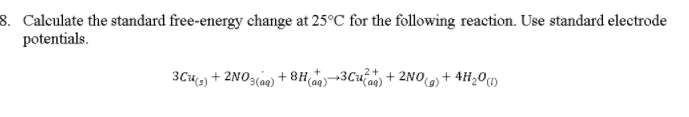# Question & Answer: Calculate the standard free-energy change at 25 degree C for the following reaction…..Calculate the standard free-energy change at 25 degree C for the following reaction. Use standard electrode potentials. 3Cu_(s) + 2NO_3_(aq) + 8H_(aq)^+ rightarrow 3Cu_(aq)^2+ + 2NO_(g) + 4 H_2O_(l)

For the given reaction,

Don't use plagiarized sources. Get Your Custom Essay on
Question & Answer: Calculate the standard free-energy change at 25 degree C for the following reaction…..
GET AN ESSAY WRITTEN FOR YOU FROM AS LOW AS \$13/PAGE

At Cathode Reduction takes place.

2 NO3- (aq) + 8 H+ ——> 2 NO(g) + 4 H2O (l)

In the above reaction for N atom Oxidation state changes from +5 to +2

At anode oxidation takes place,

3 Cu (s) ——-> 3 Cu+2 (aq)

In the above reaction for Cu atom oxidation changes from 0 to +2

E0 cell = E cathode – E anode

E0 cell = 1 – 0.34

E0 cell = 0.66

we know free energy change dG = -nFE0

n = number of electrons transferd in the given reaction